﻿

### A Quantitative Characterization of Some Finite Simple Groups Through Order and Degree Pattern

#### Abstract

Let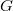$G$ be a finite group with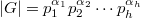$|G|=p_1^{\alpha_1}p_2^{\alpha_2}\cdots p_h^{\alpha_h}$, where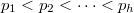$p_1 are prime numbers and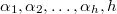$\alpha_1, \alpha_2, \ldots, \alpha_h, h$ are natural numbers. The prime graph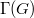$\Gamma(G)$ of$G$ is a simple graph whose vertex set is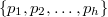$\{p_1, p_2, \ldots, p_h\}$ and two distinct primes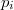$p_i$ and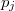$p_j$ are joined by an edge if and only if$G$ has an element of order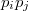$p_ip_j$. The degree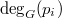${\rm deg}_G(p_i)$ of a vertex$p_i$ is the number of edges incident on$p_i$, and the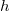$h$-tuple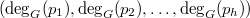$({\rm deg}_G(p_1), {\rm deg}_G(p_2), \ldots, {\rm deg}_G(p_h))$ is called the degree pattern of$G$. We say that the problem of OD-characterization is solved for a finite group$G$ if we determine the number of pairwise non-isomorphic finite groups with the same order and degree pattern as$G$. The purpose of this paper is twofold. First, it completely solves the OD-characterization problem for every finite non-Abelian simple groups their orders having prime divisors at most 17. Second, it provides a list of finite (simple) groups for which the problem of OD-characterization have been already solved.

DOI Code: 10.1285/i15900932v34n2p91

Keywords: Prime graph; degree pattern; OD-characterization

Full Text: PDFThis work is licensed under a Creative Commons Attribuzione - Non commerciale - Non opere derivate 3.0 Italia License.